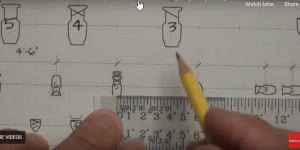# Dimensions Between Lighting UnitsIncluding lighting unit dimensions will save you a lot of time during your load in time. You don’t want to have technicians measuring with a scale ruler each time. Here I show how to use a scale rule on a pipe full of lights to add Dimensions Between Lighting Units

Would you like a PDF copy of the sheets shown in the video? Fill this out and we'll send you the download link right away via email.

We do this to avoid spam and bots. Thanks for your understanding. We'll also be keeping in touch via our newsletter which is easy to unsubscribe from if you wish.

Transcript of my video showing Dimensions Between Lighting Units:

This is our worksheet for working with a scale ruler. As you can see, it’s a sample of lighting instruments on pipes and such, and this is a standard architect scale ruler. And this is a piece of paper that has three different scale rulers to use if you don’t have an architect scale ruler. And this is available online. See the description for the links. So now the worksheet here we have several lighting units. We have several lighting pipes. We’ve got the number one electric here.

One two number three, four here, you’re going to use your 1/2″ scale here, you use your quarter inch scale. We’re going to measure the distance between the lighting units. Put the date and your name. A couple of questions to answer right there. And then a column to fill in the quantities of the lighting units on this little sample Light plot. We have a key here and these symbols tell us what all these symbols mean here.

So we have here are lighting it with an X in it. Over here we have an X that stands for a 19 degree unit. This is a PAR symbol. This is what tells us that it’s a PAR and so on, the lighting units are numbered from right to left, because when we’re dealing with a plot, we deal with from stage left to stage right. So this is one, two, three, four, five and so on.

So some samples, there’s an example here of exactly how we want to write down our dimensions, the distance from the center of this light to the center of this light is one foot six inches. Now, the dimensions are always measured from where the C-Clamp is to where the C-clamp is, on center to center on a standard unit that has a single C-clamp. So that’s the distance here. One foot six and the distance between four and five is three feet.

This is in 1/2″ scale. This is 1/2″ scale there. If we put our scale ruler here on the zero here at the middle of our lighting unit number five, we’ll see that over here in the middle of number four is three feet. Zero one to three feet. So the distance is three feet. This one, we move this over with zero in the middle of the fourth unit. And the middle of the third unit is one foot six inches, one foot six inches.

So if we take the example on different pipe, we’re going to move to the quarter-inch symbols. You see how these are much smaller than these symbols. They’re actually half the size this we’re using a quarter inch square. And in this case, we’re in our quarter and scale. So I’m going to fold the piece of paper and lay it down right here. And measure the distance between this unit and this unit to put a center one, two, three, four, five, six feet.

So we’re right here, six feet dash, zero inches. And if you forget how to do this, there are examples on the sheet. These are also positioned at distances. This is three feet, which you’ll notice that this is three feet, but so is this one. So the distance between these two is three feet in 1/2″ scale and the distance between these two is three feet 1/4″ scale. So if you take a quarter inch, put it here between these two lines, which is for these two units.

We have one, two, three, four, four feet, six inches distance. Using our quarter inch scale ruler here, we have a zero, two, four, six, which means that this is one foot, two foot three feet, four feet and so on. So here I lay this right down here. the distance from this light, that light moving up to these reference lines there, is zero, one, two, three, four, six inches because it’s between those two or more accurately, if I move it over to the left line at the four feet, this mark, four, three, two, one, zero.

And then we use the small lines here. And right here in the middle is the six inch line. Same thing happens with the half inch scale, but I have to turn the ruler over. Here we’ve got zero, one foot, two feet, three feet. For here, we put the one foot mark here, zero and then six inches right in the middle of here. And that’s how we use our scale or on our worksheet. To use the whole worksheet as a practice sheet, simply measure the distances between all the units, use the appropriate scales and write the number in just like I did here, use a nice, neat lettering and keep it clean.